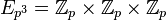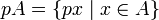# Second cohomology group for trivial group action of elementary abelian group of prime-square order on cyclic group of prime-square order

## Description of the group

Suppose$p$ is a prime number.

We consider here the second cohomology group for trivial group action$\! H^2(G,A)$

where$G$ is the elementary abelian group of prime-square order$E_{p^2} = \mathbb{Z}_p \times \mathbb{Z}_p$ and$A$ is the cyclic group of prime-square order$\mathbb{Z}_{p^2}$.

The group is isomorphic to elementary abelian group of prime-cube order$E_{p^3} = \mathbb{Z}_p \times \mathbb{Z}_p \times \mathbb{Z}_p$.

## Computation in terms of group cohomology

The cohomology group can be computed as an abstract group using the group cohomology of elementary abelian group of prime-square order, which in turn can be computed using the Kunneth formula for group cohomology combined with the group cohomology of finite cyclic groups.

We explain here the part of the computation based on the group cohomology of elementary abelian group of prime-square order. As per that page, we have:$H^2(G;A) = (\operatorname{Ann}_A(p)) \oplus (A/pA)^2$

Here,$A/pA$ is the quotient of$A$ by$pA = \{ px \mid x \in A \}$ and$\operatorname{Ann}_A(p) = \{ x \in A \mid px = 0 \}$.

In our case,$A = \mathbb{Z}/p^2\mathbb{Z}$, so we get that both$A/pA$ and$\operatorname{Ann}_A(p)$ are both isomorphic to$\mathbb{Z}/p^2\mathbb{Z}$. Plugging in, we get:$H^2(G;A) = \mathbb{Z}/p\mathbb{Z} \oplus (\mathbb{Z}/p\mathbb{Z})^2 = (\mathbb{Z}/p\mathbb{Z})^3$

which is the elementary abelian group of order$p^3$.

## Elements

### Summary

FACTS TO CHECK AGAINST (second cohomology group for trivial group action):
Background reading on relationship with extension groups: Group extension problem
Arithmetic functions of extension group:
order (thus all extension groups have the same order): order of extension group is product of order of normal subgroup and quotient group
nilpotency class: nilpotency class of extension group is between nilpotency class of quotient group and one more for central extension
derived length: derived length of extension group is bounded by sum of derived length of normal subgroup and quotient group
minimum size of generating set: minimum size of generating set of extension group is bounded by sum of minimum size of generating set of normal subgroup and quotient group|minimum size of generating set of quotient group is at most minimum size of generating set of group
WHAT'S THE TABLE BELOW?: Recall that there is a correspondence:
Elements of the group$H^2(G;A)$ for the trivial group action$\leftrightarrow$ congruence classes of central extensions with the specified subgroup$A$ and quotient group$G$.
This descends to a correspondence:
Orbits for the group action of$\operatorname{Aut}(G) \times \operatorname{Aut}(A)$ on$H^2(G;A)$$\leftrightarrow$ pseudo-congruence classes of central extensions.
The table below breaks down the second cohomology group as a union of these orbits, with (as a general rule) each row describing one orbit, i.e., one "cohomology class type", aka one "pseudo-congruence class" of central extensions. The number of rows is the number of pseudo-congruence classes of central extensions.

As mentioned earlier, the information here does not apply to the case$p = 2$. For the case$p = 2$, see second cohomology group for trivial group action of V4 on Z4.

Cohomology class type Number of cohomology classes Corresponding group extension GAP ID (second part, order is$p^3$) Stem extension? Base characteristic in whole group? Nilpotency class of whole group (at least 1, at most 2) Derived length of whole group (at least 1, at most 2) Minimum size of generating set of whole group (at least 2, at most 3) Subgroup information on base in whole group
trivial 1 direct product of cyclic group of prime-square order and elementary abelian group of prime-square order 5 No No 1 1 3
symmetric and nontrivial$p^2 - 1$ direct product of cyclic group of prime-cube order and cyclic group of prime order 2 No Yes 1 1 2
non-symmetric$p - 1$ SmallGroup(p^4,14) 3 Yes Yes 2 2 2
non-symmetric$(p - 1)(p^2 - 1)$ SmallGroup(p^4,6) 4 Yes Yes 2 2 2
Total$p^3$ (equals order of the cohomology group) -- -- -- -- -- -- -- --